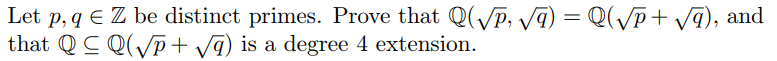Let p, q E Z be distinct primes. Prove that Q(YP,that QC Q(Vp+ Vq) is a degree 4 extension.) = Q(YP + Vq), and

Questionhelp_outlineImage TranscriptioncloseLet p, q E Z be distinct primes. Prove that Q(YP, that QC Q(Vp+ Vq) is a degree 4 extension. ) = Q(YP + Vq), and fullscreen
Step 1

To prove the statements about the given field extensions, (two quadratic and one 4th degree over Q)

Step 2

Required to prove statements 1) and 2). Note that 2) follows immediately from 1) once we show deg K =4

Step 3

1) Proof that K is co...

Want to see the full answer?

See Solution

Want to see this answer and more?

Our solutions are written by experts, many with advanced degrees, and available 24/7

See Solution
Tagged in

Math